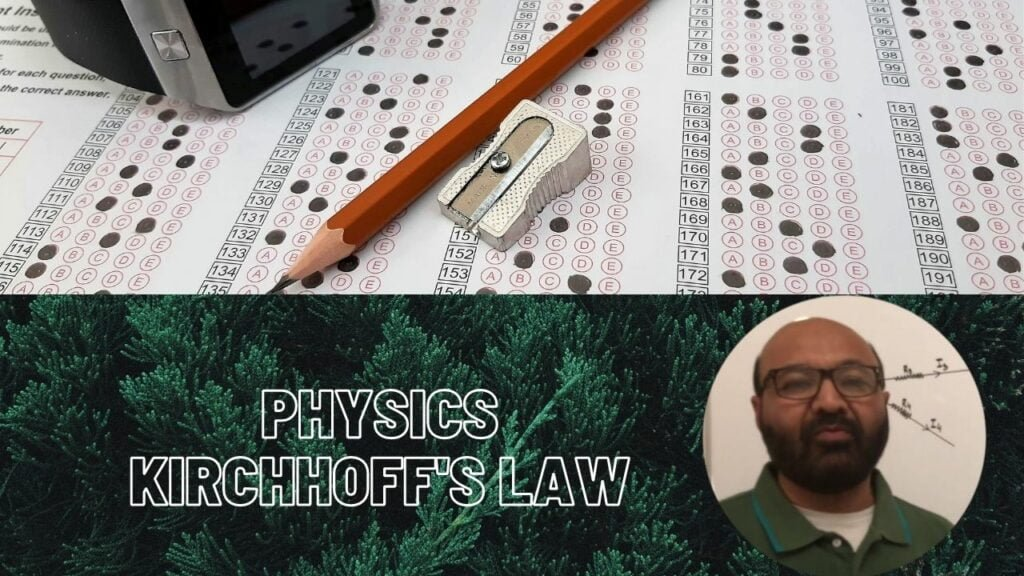Physics Lectures### Kirchhoff’s Laws

It is relatively easy to find a single equivalent resistance when two or more resistors are connected in series, parallel, or combination of both. These circuits follow Ohm’s law. However, sometimes in complex circuits, we cannot easily use Ohm’s law alone to find voltage or current in the circuit. For such complex circuits, there are laws generally called Kirchhoff’s circuit laws. The first law deals with the current flowing around a closed
circuit is called Kirchhoff’s current law (KCL). While the second law deals with the voltage sources present in a closed circuit called Kirchhoff’s voltage law (KVL).
Kirchhoff current law states that the sum of all the currents meeting at a point in a circuit is zero: ? I = 0It is a convention that a current flowing towards a point is taken as positive and that flowing away from a point is taken as negative. Considering a situation where four resistors meet at a point A. The currents flowing into the point A are I1 and I2 and currents flowing away from point A are I3 and I4. According to the convention, currents I1 and I2 are positive and currents, I3, and I4 are negative. Therefore,

I1 + I2 + (-I3) + (-I4) = 0

I1 + I2 = I3 + I4

Now we can say that sum of the currents flowing towards a point is equal to the sum of current flowing away from the point. We can use KCL when analyzing parallel circuits.

Kirchhoff voltage law states that the algebraic sum of voltage change in a closed circuit must be equal to zero.VAB + VBC + VCD + VDA = 0

We can use KVL when analyzing series circuits.

In order to understand how Kirchhoff laws help in determining current and voltage in complex circuits consider the below-mentioned example:Five basic steps for applying Kirchhoff’s circuit laws

1. Label nodes in the circuit
2. Assign the current and direction of current in each branch
3. Indicate the direction of loop
4. Apply KCL and at each node and write its equations
5. Go around each loop and apply KVL

KCL At node-A I1 + I2 – I3 = 0

or l3 = I1 + I2

At node-B I3 – I1 – I2 = 0

or l3 = I1 + I2

Before applying KVL on a circuit we must keep in mind that in a circuit:

1. Going from low potential to high potential (negative to positive) or opposite to the direction of current means a rise in voltage.
2. Going from high potential to low potential or in the direction of current means a drop in voltage.

KVL At loop-1 -10I1 – 40l3 + 10 = 0

10 = 10I1 + 40I3 (equation-1)

At loop-2 -20I2 – 40I3 + 20 = 0

20 = 20I2 + 40I3 (equation-2)

From KCL we know that I3 = I1 + I2 hence we can write equation-1 as

10 = 10I1 + 40(I1 + I2)

10 = 50I1 + 40I2 (equation-3)

Also, we can write equation-2 as

20 = 20I2 + 40(I1 + I2)

20 = 40I1 + 60I2 (equation-4)

By using simultaneous linear equation method we multiply equation-3 by 3 and equation-4 by 2

30 = 150I1 + 120I2 (equation-5)

40 = 80I1 + 120I2 (equation-6)

Tags :